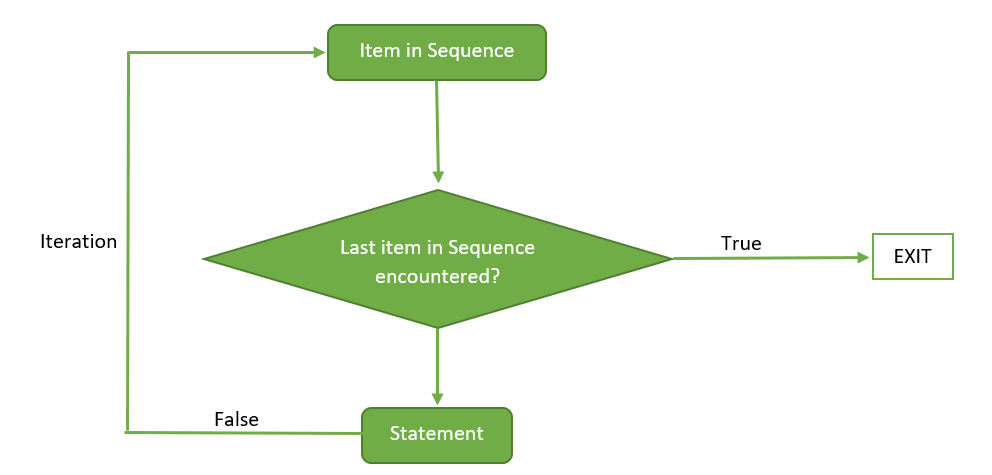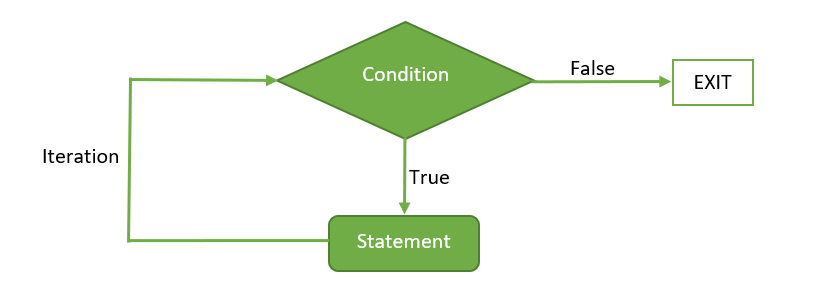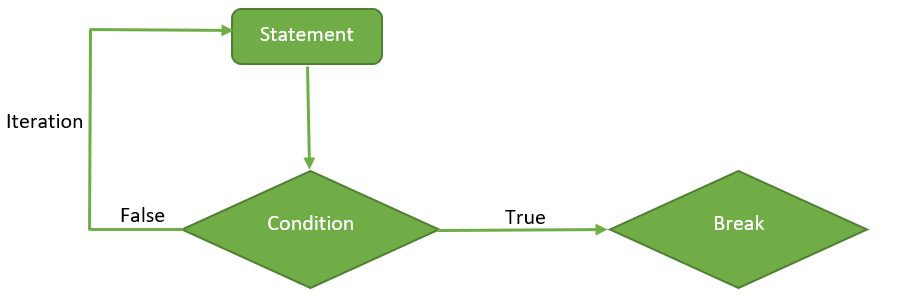# Loops in R (for, while, repeat)

• Difficulty Level : Basic
• Last Updated : 21 Oct, 2021

In R programming, we require a control structure to run a block of code multiple times. Loops come in the class of the most fundamental and strong programming concepts. A loop is a control statement that allows multiple executions of a statement or a set of statements. The word ‘looping’ means cycling or iterating.

A loop asks a query, in the loop structure. If the answer to that query requires an action, it will be executed. The same query is asked again and again until further action is taken. Any time the query is asked in the loop, it is known as an iteration of the loop. There are two components of a loop, the control statement, and the loop body.  The control statement controls the execution of statements depending on the condition and the loop body consists of the set of statements to be executed.

In order to execute the identical lines of code numerous times in a program, a programmer can simply use a loop.

• For Loop
• While Loop
• Repeat Loop

## For Loop in R

It is a type of control statement that enables one to easily construct a loop that has to run statements or a set of statements multiple times. For loop is commonly used to iterate over items of a sequence. It is an entry controlled loop, in this loop the test condition is tested first, then the body of the loop is executed, the loop body would not be executed if the test condition is false.

### R – For loop Syntax:

```for (value in sequence)
{
statement
}```

### For Loop Flow Diagram:Below are some programs to illustrate the use of for loop in R programming.

Example 1: Program to display numbers from 1 to 5 using for loop in R.

## R

 `# R program to demonstrate the use of for loop` `# using for loop``for ``(val ``in` `1: 5)``{``    ``# statement``    ``print``(val)``}`

Output:

``` 1
 2
 3
 4
 5```

Here, for loop is iterated over a sequence having numbers from 1 to 5. In each iteration, each item of the sequence is displayed.

Example 2: Program to display days of a week.

## R

 `# R program to illustrate``# application of for loop` `# assigning strings to the vector``week < - ``c``(``'Sunday'``,``           ``'Monday'``,``           ``'Tuesday'``,``           ``'Wednesday'``,``           ``'Thursday'``,``           ``'Friday'``,``           ``'Saturday'``)` `# using for loop to iterate``# over each string in the vector``for ``(day ``in` `week)``{` `    ``# displaying each string in the vector``    ``print``(day)``}`

Output:

``` "Sunday"
 "Monday"
 "Tuesday"
 "Wednesday"
 "Thusrday"
 "Friday"
 "Saturday"```

In the above program, initially, all the days(strings) of the week are assigned to the vector week. Then for loop is used to iterate over each string in a week. In each iteration, each day of the week is displayed.

## While Loop in R

It is a type of control statement which will run a statement or a set of statements repeatedly unless the given condition becomes false. It is also an entry controlled loop, in this loop the test condition is tested first, then the body of the loop is executed, the loop body would not be executed if the test condition is false.

### R – While loop Syntax:

```while ( condition )
{
statement
}```

### While loop Flow Diagram:Below are some programs to illustrate the use of the while loop in R programming.

Example 1: Program to display numbers from 1 to 5 using while loop in R.

## R

 `# R program to demonstrate the use of while loop` `val = 1` `# using while loop``while ``(val <= 5)``{``    ``# statements``    ``print``(val)``    ``val = val + 1``}`

Output:

``` 1
 2
 3
 4
 5```

Initially, the variable value is initialized to 1. In each iteration of the while loop the condition is checked and the value of val is displayed and then it is incremented until it becomes 5 and the condition becomes false, the loop is terminated.

Example 2: Program to calculate factorial of a number.

## R

 `# R program to illustrate``# application of while loop` `# assigning value to the variable``# whose factorial will be calculated``n < - 5` `# assigning the factorial variable``# and iteration variable to 1``factorial < - 1``i < - 1` `# using while loop``while ``(i <= n)``{` `    ``# multiplying the factorial variable``    ``# with the iteration variable``    ``factorial = factorial * i` `    ``# incrementing the iteration variable``    ``i = i + 1``}` `# displaying the factorial``print``(factorial)`

Output:

` 120`

Here, at first, the variable n is assigned to 5 whose factorial is going to be calculated, then variable i and factorial are assigned to 1. i will be used for iterating over the loop, and factorial will be used for calculating the factorial. In each iteration of the loop, the condition is checked i.e. i should be less than or equal to 5, and after that factorial is multiplied with the value of i, then i is incremented. When i becomes 5, the loop is terminated and the factorial of 5 i.e. 120 is displayed beyond the scope of the loop.

## Repeat Loop in R

It is a simple loop that will run the same statement or a group of statements repeatedly until the stop condition has been encountered. Repeat loop does not have any condition to terminate the loop, a programmer must specifically place a condition within the loop’s body and use the declaration of a break statement to terminate this loop. If no condition is present in the body of the repeat loop then it will iterate infinitely.

### R – Repeat loop Syntax:

```repeat
{
statement

if( condition )
{
break
}
}```

### Repeat loop Flow Diagram:To terminate the repeat loop, we use a jump statement that is the break keyword. Below are some programs to illustrate the use of repeat loops in R programming.

Example 1: Program to display numbers from 1 to 5 using repeat loop in R.

## R

 `# R program to demonstrate the use of repeat loop` `val = 1` `# using repeat loop``repeat``{``    ``# statements``    ``print``(val)``    ``val = val + 1` `    ``# checking stop condition``    ``if``(val > 5)``    ``{``        ``# using break statement``        ``# to terminate the loop``        ``break``    ``}``}`

Output:

``` 1
 2
 3
 4
 5```

In the above program, the variable val is initialized to 1, then in each iteration of the repeat loop the value of val is displayed and then it is incremented until it becomes greater than 5. If the value of val becomes greater than 5 then break statement is used to terminate the loop.

Example 2: Program to display a statement five times.

## R

 `# R program to illustrate``# the application of repeat loop` `# initializing the iteration variable with 0``i < - 0` `# using repeat loop``repeat``{``    ``# statement to be executed multiple times``    ``print``(``"Geeks 4 geeks!"``)` `    ``# incrementing the iteration variable``    ``i = i + 1` `    ``# checking the stop condition``    ``if ``(i == 5)``    ``{``        ``# using break statement``        ``# to terminate the loop``        ``break``    ``}``}`

Output:

``` "Geeks 4 geeks!"
 "Geeks 4 geeks!"
 "Geeks 4 geeks!"
 "Geeks 4 geeks!"
 "Geeks 4 geeks!"```

Here, initially the variable i is initialized with 0 then in each iteration of the repeat loop after printing Geeks 4 geeks! the value of i is incremented till it becomes 5 and the condition in the if statement becomes true then, the break statement is executed to terminate the repeat loop.

## Jump Statements in Loop

We use a jump statement in loops to terminate the loop at a particular iteration or to skip a particular iteration in the loop. The two most commonly used jump statements in loops are:

• Break Statement: The break keyword is a jump statement that is used to terminate the loop at a particular iteration.

Example:

## R

 `# R program to illustrate``# the use of break statement` `# using for loop``# to iterate over a sequence``for ``(val ``in` `1: 5)``{``    ``# checking condition``    ``if ``(val == 3)``    ``{``        ``# using break keyword``        ``break``    ``}` `    ``# displaying items in the sequence``    ``print``(val)``}`

Output:

``` 1
 2```

In the above program, if the value of val becomes 3 then the break statement will be executed and the loop will terminate.

• Next Statement: The next keyword is a jump statement which is used to skip a particular iteration in the loop.

Example:

## R

 `# R program to illustrate``# the use of next statement` `# using for loop``# to iterate over the sequence``for ``(val ``in` `1: 5)``{``    ``# checking condition``    ``if ``(val == 3)``    ``{``        ``# using next keyword``        ``next``    ``}` `    ``# displaying items in the sequence``    ``print``(val)``}`

Output:

``` 1
 2
 4
 5```

In the above program, if the value of Val becomes 3 then the next statement will be executed hence the current iteration of the loop will be skipped. So 3 is not displayed in the output.

As we can conclude from the above two programs the basic difference between the two jump statements is that the break statement terminates the loop and the next statement skips a particular iteration of the loop.

My Personal Notes arrow_drop_up